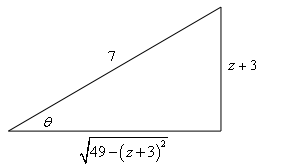Paul's Online Notes
Home / Calculus II / Integration Techniques / Trig Substitutions
Show Mobile Notice Show All Notes Hide All Notes
Mobile Notice
You appear to be on a device with a "narrow" screen width (i.e. you are probably on a mobile phone). Due to the nature of the mathematics on this site it is best views in landscape mode. If your device is not in landscape mode many of the equations will run off the side of your device (should be able to scroll to see them) and some of the menu items will be cut off due to the narrow screen width.

### Section 7.3 : Trig Substitutions

15. Use a trig substitution to evaluate $$\displaystyle \int{{\frac{{{{\left( {z + 3} \right)}^5}}}{{{{\left( {40 - 6z - {z^2}} \right)}^{\frac{3}{2}}}}}\,dz}}$$.

Show All Steps Hide All Steps

Start Solution

The first thing we’ll need to do here is complete the square on the polynomial to get this into a form we can use a trig substitution on.

\begin{align*}40 - 6z - {z^2} & = - \left( {{z^2} + 6z - 40} \right) = - \left( {{z^2} + 6z + 9 - 9 - 40} \right) = - \left[ {{{\left( {z + 3} \right)}^2} - 49} \right]\\ & = 49 - {\left( {z + 3} \right)^2}\end{align*}

The integral is now,

$\int{{\frac{{{{\left( {z + 3} \right)}^5}}}{{{{\left( {40 - 6z - {z^2}} \right)}^{\frac{3}{2}}}}}\,dz}} = \int{{\frac{{{{\left( {z + 3} \right)}^5}}}{{{{\left( {49 - {{\left( {z + 3} \right)}^2}} \right)}^{\frac{3}{2}}}}}\,dz}}$

Now we can proceed with the trig substitution.

Show Step 2

It looks like we’ll need to the following trig substitution.

$z + 3 = 7\sin \left( \theta \right)$

Next let’s eliminate the root.

${\left( {49 - {{\left( {z + 3} \right)}^2}} \right)^{\frac{3}{2}}} = {\left[ {\sqrt {49 - \left( {z + 3} \right)} } \right]^3} = {\left[ {\sqrt {49 - 49{{\sin }^2}\left( \theta \right)} } \right]^3} = {\left[ {7\sqrt {{{\cos }^2}\left( \theta \right)} } \right]^3} = 343{\left| {\cos \left( \theta \right)} \right|^3}$

Next, because we are doing an indefinite integral we will assume that the cosine is positive and so we can drop the absolute value bars to get,

${\left( {49 - {{\left( {z + 3} \right)}^2}} \right)^{\frac{3}{2}}} = 343{\cos ^3}\left( \theta \right)$

For a final substitution preparation step let’s also compute the differential so we don’t forget to use that in the substitution!

$\left( 1 \right)dz = 7\cos \left( \theta \right)\,d\theta \hspace{0.25in}\hspace{0.25in} \Rightarrow \hspace{0.25in}\hspace{0.25in}dz = 7\cos \left( \theta \right)\,d\theta$

Recall that all we really need to do here is compute the differential for both the right and left sides of the substitution.

Show Step 3

Now let’s do the actual substitution.

$\int{{\frac{{{{\left( {z + 3} \right)}^5}}}{{{{\left( {40 - 6z - {z^2}} \right)}^{\frac{3}{2}}}}}\,dz}} = \int{{\frac{{16807{{\sin }^5}\left( \theta \right)}}{{343{{\cos }^3}\left( \theta \right)}}\left( {7\cos \left( \theta \right)} \right)\,d\theta }} = 343\int{{\frac{{{{\sin }^5}\left( \theta \right)}}{{{{\cos }^2}\left( \theta \right)}}\,d\theta }}$

Do not forget to substitute in the differential we computed in the previous step. This is probably the most common mistake with trig substitutions. Forgetting the differential can substantially change the problem, often making the integral very difficult to evaluate.

Show Step 4

We now need to evaluate the integral. Here is that work.

\begin{align*}\int{{\frac{{{{\left( {z + 3} \right)}^5}}}{{{{\left( {40 - 6z - {z^2}} \right)}^{\frac{3}{2}}}}}\,dz}} & = 343\int{{\frac{{{{\left[ {1 - {{\cos }^2}\left( \theta \right)} \right]}^2}}}{{{{\cos }^2}\left( \theta \right)}}\sin \left( \theta \right)\,d\theta }}\hspace{0.25in}\hspace{0.25in}u = \cos \left( \theta \right)\\ & = - 343\int{{\frac{{{{\left[ {1 - {u^2}} \right]}^2}}}{{{u^2}}}\,du}} = - 343\int{{{u^{ - 2}} - 2 + {u^2}\,du}}\\ & = - 343\left( { - {u^{ - 1}} - 2u + \frac{1}{3}{u^3}} \right) + c\\ & = - 343\left( { - \frac{1}{{\cos \left( \theta \right)}} - 2\cos \left( \theta \right) + \frac{1}{3}{{\cos }^3}\left( \theta \right)} \right) + c\end{align*}

Don’t forget all the “standard” manipulations of the integrand that we often need to do in order to evaluate integrals involving trig functions. If you don’t recall them you’ll need to go back to the previous section and work some practice problems to get good at them.

Every trig substitution problem reduces down to an integral involving trig functions and the majority of them will need some manipulation of the integrand in order to evaluate.

Show Step 5

As the final step we just need to go back to $$z$$’s. To do this we’ll need a quick right triangle. Here is that work.

 From the substitution we have, $\sin \left( \theta \right) = \frac{{z + 3}}{7}\,\,\,\,\,\left( { = \frac{{{\mbox{adj}}}}{{{\mbox{hyp}}}}} \right)$ From the right triangle we get, $\cos \left( \theta \right) = \frac{{\sqrt {49 - {{\left( {z + 3} \right)}^2}} }}{7}$The integral is then,

$\int{{\frac{{{{\left( {z + 3} \right)}^5}}}{{{{\left( {40 - 6z - {z^2}} \right)}^{\frac{3}{2}}}}}\,dz}} = \require{bbox} \bbox[2pt,border:1px solid black]{{\frac{{2401}}{{\sqrt {49 - {{\left( {z + 3} \right)}^2}} }} + 98\sqrt {49 - {{\left( {z + 3} \right)}^2}} - \frac{{{{\left( {49 - {{\left( {z + 3} \right)}^2}} \right)}^{\frac{3}{2}}}}}{3} + c}}$